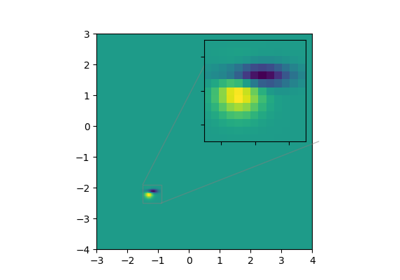# matplotlib.axes.Axes.inset_axes¶

`Axes.``inset_axes`(bounds, *, transform=None, zorder=5, **kwargs)[source]

Add a child inset axes to this existing axes.

Parameters: bounds : [x0, y0, width, height] Lower-left corner of inset axes, and its width and height. transform : `Transform` Defaults to `ax.transAxes`, i.e. the units of rect are in axes-relative coordinates. zorder : number Defaults to 5 (same as `Axes.legend`). Adjust higher or lower to change whether it is above or below data plotted on the parent axes. **kwargs Other kwargs are passed on to the `axes.Axes` child axes. Axes The created `axes.Axes` instance.

Warning

This method is experimental as of 3.0, and the API may change.

Examples

This example makes two inset axes, the first is in axes-relative coordinates, and the second in data-coordinates:

```fig, ax = plt.suplots()
ax.plot(range(10))
axin1 = ax.inset_axes([0.8, 0.1, 0.15, 0.15])
axin2 = ax.inset_axes(
[5, 7, 2.3, 2.3], transform=ax.transData)
```

## Examples using `matplotlib.axes.Axes.inset_axes`¶Zoom region inset axes